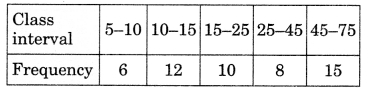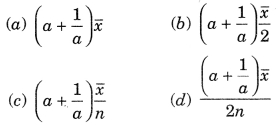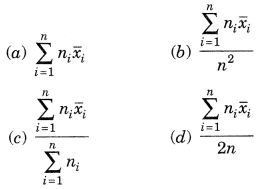Check the below Online Education NCERT MCQ Questions for Class 9 Maths Chapter 14 Statistics with Answers Pdf free download. MCQ Questions for Class 9 Maths with Answers were prepared based on the latest exam pattern. We have provided Statistics Class 10 Maths MCQs Questions with Answers to help students understand the concept very well. https://ncertmcq.com/mcq-questions-for-class-9-maths-with-answers/

Students can also refer to NCERT Solutions for Class 9 Maths Chapter 14 Statistics for better exam preparation and score more marks.

## Statistics Class 9 MCQs Questions with Answers

Statistics Class 9 MCQ Chapter 14 Question 1.
The class mark of the class 90-130 is:
(a) 90
(b) 105
(c) 115
(d) 110

Statistics MCQ Class 9 Chapter 14 Question 2.
The range of the data:
25, 81, 20, 22, 16, 6, 17,15,12, 30, 32, 10, 91, 8, 11, 20 is
(a) 10
(b) 75
(c) 85
(d) 26

Class 9 Statistics MCQ Chapter 14 Question 3.
In a frequency distribution, the mid value of a class is 10 and the width of the class is 6. The upper limit of the class is:
(a) 6
(b) 7
(c) 10
(d) 13Statistics Class 9 MCQ With Answers Chapter 14 Question 4.
The width of each of five continuous classes in a frequency distribution is 5 and the lower class-limit of the lowest class is 10. The lower class-limit of the highest class is:
(a) 15
(b) 30
(c) 35
(d) 40

Class 9 Maths Chapter 14 MCQ Question 5.
Let m be the mid-point and 1 be the lower class limit of a class in a continuous frequency distribution. The upper class limit of the class is:
(a) 2m + l
(b) 2m – l
(c) m – l
(d) m – 2l

MCQ On Statistics Class 9 Chapter 14 Question 6.
The class marks of a frequency distribution are given as follows:
15, 20, 25, …
The class corresponding to the class mark 15 is:
(a) 12.5 – 17.5
(b) 17.5 – 22.5
(c) 18.5 – 21.5
(d) 19.5 – 20.5

MCQ Of Statistics Class 9 Chapter 14 Question 7.
In the class intervals 10-20, 20-30, the number 20 is included in:
(a) 10-20
(b) 20-30
(c) both the intervals
(d) none of these intervals

Ch 14 Maths Class 9 MCQ Question 8.
A grouped frequency table with class intervals of equal sizes using 250-270 (270 not included in this interval) as one of the class interval is constructed for the following data:
268, 220, 368, 258, 242, 310, 272, 342, 310, 290, 300, 320, 319, 304,402, 318, 406, 292, 354, 278, 210, 240, 330, 316, 406, 215, 258, 236.
The frequency of the class 370-390 is:
(a) 0
(b) 1
(c) 3
(d) 5

Class 9 Maths Ch 14 MCQ Question 9.
A grouped frequency distribution table with classes of equal sizes using 63-72 (72 included) as one of the class is constructed for the following data:
30, 32, 45, 54, 74, 78, 108, 112, 66, 76, 88, 40, 14, 20, 15, 35, 44, 66, 75, 84, 95, 96, 102, 110, 88, 74, 112, 14, 34, 44.
The number of classes in the distribution will be:
(a) 9
(b) 10
(c) 11
(d) 12

Chapter 14 Maths Class 9 MCQ Question 10.
To draw a histogram to represent the following frequency distribution:the adjusted frequency for the class 25-45 is:
(a) 6
(b) 5
(c) 3
(d) 2

MCQ Questions For Class 9 Maths Statistics Chapter 14 Question 11.
The mean of five numbers is 30. If one number is excluded, their mean becomes 28. the excluded number is:
(a) 28
(b) 30
(c) 35
(d) 38

Class 9 Maths Statistics MCQs Chapter 14 Question 12.
If $$\bar { x }$$ represents the mean of n observations x1, x2, …, xn, then value of $$\sum_{i=1}^{n}\left(x_{i}-\bar{x}\right)$$ is:
(a) -1
(b) 0
(c) 1
(d) n – 1

MCQs Statistics Class 9 Chapter 14 Question 13.
If each observation of the data is increased by 5, then their mean
(a) remains the same
(b) becomes 5 times the original mean
(c) is decreased by 5
(d) is increased by 5

Answer: (d) is increased by 5

Class 9 Maths Chapter 14 MCQ With Answers Question 14.
Let $$\bar { x }$$ be the mean of x1, x2, …, xn and y the mean of y1, y2, …, yn. If z is the mean of x1, x2, …. xn, y1, y2, …, yn, then z is equal to
(a) $$\bar {x}+\bar{y}$$
(b) $$\frac{\bar {x}+\bar{y}}{2}$$
(c) $$\frac{\bar {x}+\bar{y}}{n}$$
(d) $$\frac{\bar {x}+\bar{y}}{2n}$$

Answer: (b) $$\frac{\bar {x}+\bar{y}}{2}$$

Class 9 Maths Statistics MCQ Chapter 14 Question 15.
If $$\bar { x }$$ is the mean of x1, x2, …, xn, then for a ≠ 0, the mean of ax1, ax2, …, axn, $$\frac{x_1}{a}$$, $$\frac{x_2}{a}$$, …….., $$\frac{x_n}{a}$$ isStatics Class 9th MCQ Chapter 14 Question 16.
If $$\bar { x_1 }$$, $$\bar { x_2 }$$, $$\bar { x_3 }$$, …….., $$\bar { x_n }$$ are the means of n groups with n1, n2, ……. nn number of observations respectively, then the mean x of all the groups taken together is given by:Class 9 Ch 14 Maths MCQ Question 17.
The median of the data
78, 56, 22, 34, 45, 54, 39, 68, 54, 84 is
(a) 45
(b) 49.5
(c) 54
(d) 56Class 9 Chapter 14 Maths MCQ Question 18.
For drawing a frequency polygon of a continous frequency distribution, we plot the points whose ordinates are the frequencies of the respective classes and abcissae are respectively:
(a) upper limits of the classes
(b) lower limits of the classes
(c) class marks of the classes
(d) upper limits of preceding classes

Answer: (c) class marks of the classes

MCQ Of Ch 14 Maths Class 9 Question 19.
Mode of the data
15, 14, 19, 20, 16, 15, 16, 14, 15, 18, 14, 19, 16, 17, 16 is
(a) 14
(b) 15
(c) 16
(d) 17

Ch 14 Class 9 Maths MCQ Question 20.
The mean of 25 observations is 26. Out of these observations if the mean of first 13 observations is 22 and that of the last 13 observations is 30, the 13th observation is:
(a) 23
(b) 26
(c) 28
(d) 30